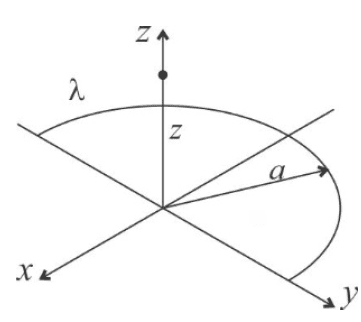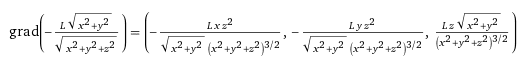# Electric Field from its Potential of a Half Circle along its Z axis

AndresPB
Homework Statement:
Find the Electric Potential Energy of half a circle charged with a Linear Charge Density: "Lambda" along its z axis. After that, find the Electric Field "E" deriving its potential energy.
Relevant Equations:
Potential Energy dV = (1/(4*Pi*Epsilon_0))*(dQ/|r-r'|)
Electric Field E = - ∇ VSo I figured out the potential is: dV = (1/(4*Pi*Epsilon_0))*[λ dl/sqrt(z^2+a^2)]
.
From that expression: We can figure out that since its half a ring we have to integrate from 0 to pi*a, so we would get:

V = (1/(4*Pi*Epsilon_0))*[λ {pi*a]/sqrt(z^2+a^2)]

In that expression: a = sqrt(x^2+y^2)

What I am having trouble is getting the electric field from this expression. When I do ∇ V, I am getting three components for my electric field in the (x,y,z), directions. But when I analyze the problem. It is clear to me that there is not a "y" component, as there is simetry along this axis. What shall I do?

The answer I am getting at the moment is this ("L" in this case is: (1/(4*Pi*Epsilon_0))*[λ {pi}]):Last edited: